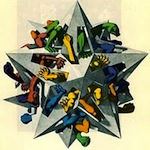# How to generate a Recursive Fractal.Fractals have been commonly known by its appearance or artistic expression. Benoît Mandelbrot defended its importance now begins to glimpse. Escher the drew from his imagination, without knowing complex equations representing.

(Imagen M.C. Escher's "Gravity")

The use of fractals in diversss disciplines, as generators of complex systems models, are an area of ​​research ever more present.

An approach to fractal geometry can be easily performed by the Koch curve.

## Koch Curve

The Koch curve, also known as snowflake is a fractal that can be obtained by different methods as so-called IFS or Function Systems iterated (Deterministic or), rule-based systems, etc..

The recursive algorithm has also the virtue of representing a concept closely associated with fractals: infinity. The essence of recursion to describe a very simple form of the curve itself. A universe that contains another and this in turn copy the pattern on a smaller scale (so contractive) an endlessly repeating sequence.

Koch curve belongs to the self-similar fractal, be the method of obtaining the deterministic.

## Generation of Koch Curve

For determining a deterministic fractal requires a starting element called initiator, and initiator modification pattern called generator.

The initiator is subdivided into parts that are replaced by the generator in an iterative process and infinite.

Koch curve taken as initiator straight line segment.

The generator divided into three equal parts segment, eliminates the central section and adds two, in his stead, equal dimensions. The angles correspond to an equilateral triangle.

The process is repeated recursively, applying the generator to each of the resulting segments.

## Fractal Dimension

The dimension an object is placed or topological concept that classifies objects metric spaces. The intuitive notion of whole dimensions spaces clashes with the so-called fractal dimensions, taking actual values.

The Peano curve is a curve capable of filling up. Do you have therefore two dimensions?, one wonders.

Is associated with a fractal dimension of roughness, or fragmentation, thereof, so that a greater dimension present a more rough or jagged. In any case gives information about its complexity characterizing.

Different calculation methods  of the fractal dimensions, as the Hausdorff dimension, Internal similarity, Bouligand, Kolmogoroff ...It can be set basing on iterations or divisions analogies Euclidean Space:

By dividing the sides of a cube by its midpoint, can be determined n= 8 identical cubes length sides half the original.

The scaling factor s= 1/2 permite relate to the value n such that:

n.sD=1

being the value of the variable D the dimension of the object.

Similarly by dividing a square n=4 equal, fulfills the relation s=1/2 ,and in this case D=2 the dimension of the object.

Koch curve has a ratio s = 1/3, with n = 4, so its fractal dimension is:

D=ln4/ln3 ~ 1.269

## Autosemejanza

Repeating patterns of these fractal topological (at different scales) lead to call them self-similar.

Contain parts which are smaller versions of the whole object size.

In the case of random variations can be applied to small scale subparts, is said to be statistically self-similar fractal.

Koch curve can be generated, in each iteration, repeating four times the pattern generator exposed.

The figure has highlighted one of the repeated elements for determining the second iteration. Moving and copying the appropriate scale generator may be generating the different steps or iterations in generating process.

 The function takes two parameters that identify the initiator line and report the number of the depth of recursion to perform. At the beginning of the execution checks whether the condition stop, namely, whether we should call the function again. If the last iteration line is painted If NO Divide the line in the four sections needed Call function recursively for each of the sections, reducing the number of iterations calculating pending. Función_Pinta_Koch_Recursivo(Linea2D,NumIteraciones)

To calculate the new segment is generated from any one AB, determining the coordinates of the same in the following manner.The points C and D are obtained by similarity, being the corresponding coordinates :

Ci = Ai + (Two-Ai)/3; y Di = Bi – (Two-Ai)/3;

The point E is on the symmetry axis of the figure, at a distance H on the segment AB and a perpendicular from the midpoint.

You can also find turning 60 point D with center C.

## Fractals in art

Several studies have used very artistic consciously or not, geometric design structures whose essence is obtained in fractals.

The most famous lines are computer-generated representations seeking ways to Colorists, with three-dimensional depth, from different algorithms.

Other artists have worked with traditional media yet, representation of thought looking through the union of artistic graphics and geometry studies.

Notably, the work of M. C. Escher en su serie "Gravity", "Double Planet" etc.., where you can find Kepler Fractals  and .

They take other forms as initiators (pentagram)or three dimensions

Recursive Fractals: Koch Curve [JAVA]

 FRACTALS Non-Integral Dimensions and Applications. John Wiley & Sons. University of Paris VII
 Graphics by computadora with OpenGL. Donald Eran. Pearson Prentice Hall
”Computers and Graphics” Flight. 19, Not. 6, pp. 885-888, 1995

One of the existing classifications divides them into self-similar (Losstatistically self-similar Set the model trees, shrubs and other plants), Autoafines (Los statistically self-affine for defining land, water, clouds etc..) Invariant sets and fractals ( which include autocuadráticos as the Mandelbrot set)